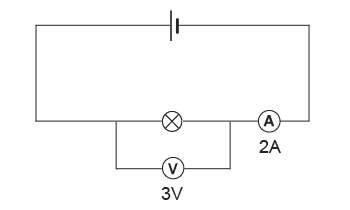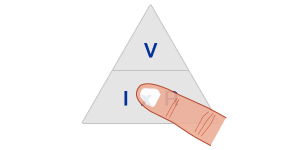## Using the Equation: V = I x R

A simple way of using the Ohm’s Law relationship is to use the triangle method. This involves taking the equation in the form where there is only multiplication and no division. In the case of Ohm’s Law in the form V = I x R and arranging it in a triangle so that V is at the top and I and R at the bottom.

## Example

In the circuit below the voltmeter reading across the lamp is 3V and the ammeter reads 2A of electrical current. What is the resistance across the lamp?Potential Difference across the lamp = V = 3V
Electrical current through the lamp = I = 2A
Electrical resistance across the lamp = R = ?

Using V = I x RRequire resistance, therefore cover R in the triangle.

Thus,
R = V / I
R = 3 / 2 = 1.5Ω